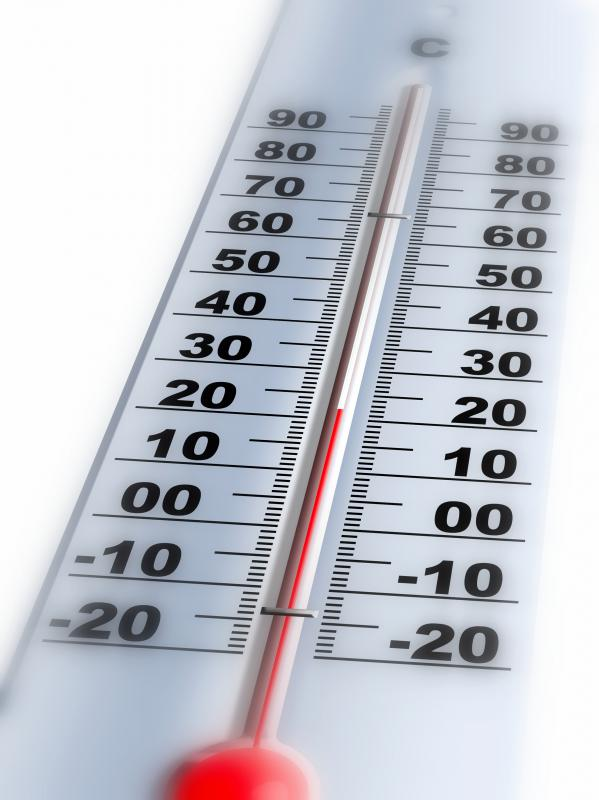# What Is Absolute Temperature? (with picture)

Vincent Summers
Vincent SummersThermometers measure absolute temperature.

Absolute temperature is the temperature measured using a scale beginning at zero, with that zero being the coldest theoretically attainable temperature in nature. There are two common absolute temperature scales derived from the Fahrenheit scale and the Celsius, or centigrade, scale. The former is the Rankine scale, and the latter is the Kelvin scale. Although still used for ordinary purposes, both the Celsius and Fahrenheit scales, with their lower-end value below zero, are less desirable for computational scientific purposes. Zero degrees Rankine is identical to zero degrees Celsius.

Simply put, temperature is an indicator of how hot or how cold an object is relative to other objects. Since temperatures vary according to season and situation, a scale complete with intermediate gradations was developed to enable comparisons. Two fixed points are needed to create a useful scale — a global, invariable standard. The logical choice upon which to base the standard temperature scales was water, since it is abundant, accessible, changes state at certain temperatures and can be readily purified. As mentioned above, however, temperature relates to heat, and heat relates at a more basic level to atomic and molecular movement.

Energy can be absorbed by atoms and molecules in a variety of ways, such as through electron excitation, the transfer of an electron from a lower to a higher orbital state. In general, however, energy is absorbed and increases the motion of the entire atom or molecule. That energy — the energy leading to "kinesis," or movement — is kinetic energy. There is an equation that ties kinetic energy to heat: E = 3/2 kT, where E is the average kinetic energy of a system, k is the Boltzmann constant and T is the absolute temperature in degrees Kelvin. Note that in this calculation, if the absolute temperature is zero, the equation indicates there is no kinetic energy or motion at all.

A kind of energy actually does still exist at zero degrees absolute temperature, even though this is not what the classical physics equation above indicates. Remaining motion is predicted by quantum mechanics and is associated with a specific type of energy called the "zero-point vibrational energy." Quantitatively, this energy can be calculated mathematically from the equation for a quantum harmonic oscillator and with knowledge of the Heisenberg Uncertainty Principle. That principle of physics dictates that it is not possible to know both the position and the momentum of very tiny particles, hence if the location is known, the particle must retain a miniscule vibrational component.

## You might also Like## Discussion Comments

Raynbow

@talentryto- I think that most digital thermometers are pretty accurate, because they use internal computerized technology to detect temperatures.

Talentryto

Since not many people use the old types of thermometers that contained mercury for safety reasons, what are the most accurate thermometers for getting true readings of accurate temperatures? It seems like different types of thermometers give different readings for the same temperatures.

Forgot password?
###### Register:
•Thermometers measure absolute temperature.